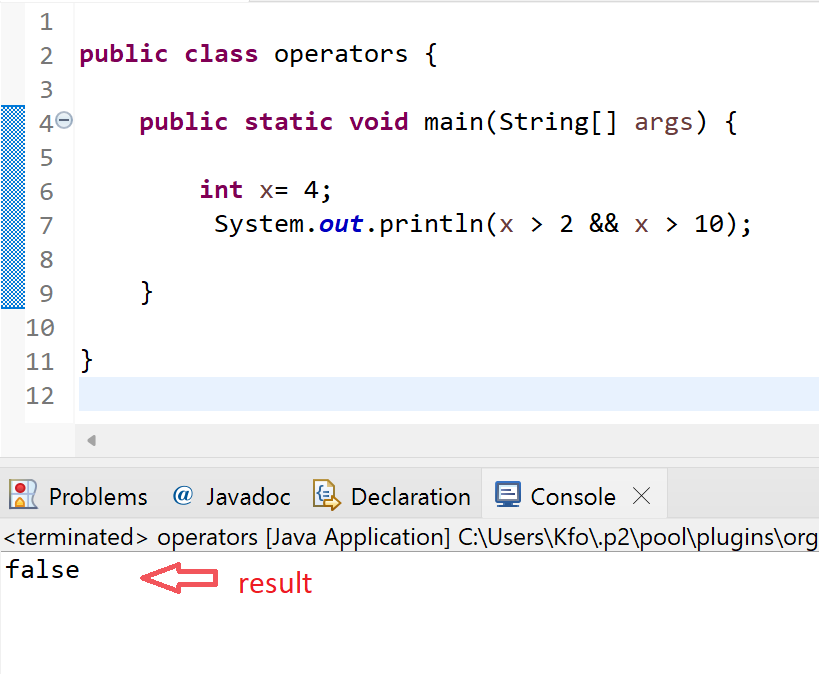•A.razaq

# T17- Logical Operators

In java we use operators to do calculations on variables or other operations such as comparison and so on , in this tutorial we will begin by logical Operators and understand them one by one.• && (conditional AND).

• || (conditional OR).

• ! (logical NOT).

&& (conditional AND)

lets begin with the first one ( && ) , this operator returns true if both statements are true, meaning we use it for two conditions and if both conditions are met it will return true if one condition only is met it returns false

lets take an example:

```
int x= 4;
System.out.println(x > 2 && x < 10);```

in this code we want to test the two conditions which are , ( x > 2) and second condition (x < 10) , in the example above the value of x is (4) which is more then 2 but less than 10 so both conditions are met

outputas we see, both conditions are met so it returns ( true )

now lets change the conditions

```int x= 4;
System.out.println(x > 2 && x > 10);```

in this example , we want x to be more than 2 and more than 10

lets test it out.now the first condition is met but the second condition was not , so it returns ( false )

||

now with the second operator || , this operator requires only one statement to be true , it returns true if one of the statements is true so only 1 condition is required to be met.

lets take an example :

```          int x= 4;
System.out.println(x > 2 || x > 10);```

in this example only one condition need to be true because the operator || is basically says ( this statement OR this statement )

lets test it out :we can see it returns true even though only one condition is met , ( x>2) which is true but ( x < 10 ) is false , but the result is true because the operator || needs only one statement to be true

lets test another case but with three conditions

``` int x= 4;
System.out.println(x > 2 || x > 10 || x < 1);```now we have 3 conditions

1. x>2 - is true

2. x>10 - is false

3. x<1- is false

and the result is true because it needs only one to be true or all to be false .

! (logical NOT)

this operator gives the opposite of the result, returns false if the result is true.

an example :

now this code from the previous example will result in ( true )

```          int x= 4;
System.out.println(x > 2 || x > 10);```

now to use the this operator we do the following by beginning a parenthesis

( ! ( statement ) ) then we close the outer parenthesis

```               int x= 4;
System.out.println( ! (x > 2 || x > 10) ) ;```as we see here the original result is true but when we use the operator ( ! ) it reverse the results so we get false.